Dr. Herrick's CH222 Pressure Quiz 7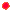Chemical Reaction Kinetics Copyright D. Herrick20 Questions from past exams. Practice for speed.  Aim for 1 minute per problem.

 Constants and formulas: exp(x) = ex 1 year = 365 days; 1 hr = 60 min; 1 min = 60 s freezing point of water = 0.00°C = 273.15K Gas constant: R = 0.08206 L-atm/mol-K = 8.314 J/mol-K Arrhenius equation:    k = A exp(-Ea/RT)     ln(k2/k1) = (Ea/R)(1/T1 - 1/T2)  first-order decay:      [C]=[C]oexp(-kt);    t1/2 = ln(2)/k

1.

Use the data for the reaction 2A + B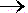C + D

 [A] (mol/L) [B] (mol/L) initial rate (mol/L-s) 0.2 0.1 5 0.2 0.2 20 0.6 0.1 15

to find the empirical rate law.

 A) rate = k[A][B] B) rate = k[A]2[B]2 C) rate = k[A][B]3/2 D) rate = k[A][B]2 E) rate = k[A]2[B]
 2 The activation energy of a reaction can be lowered by ______.
 A) raising the temperature B) removing products C) adding a catalyst D) adding more reactants E) lowering the temperature
3. Predict the overall rate law for the gas reaction consistent with the elementary mechanism
 (1) O3O2 + O (slow) (2) O + NONO2 (fast)
 A) rate = k[O3][NO] B) rate = k[NO][O3][O2]-1 C) rate = k[O][NO] D) rate = k[O3] E) rate = k[O3][NO][O]4 A first-order chemical decomposition ABA + B has a rate constant of 0.023 s-1 at 300K.  If the initial concentration of AB is 2.0 M, what is the concentration of AB after 80 s?
 A) 0.13M B) 0.54M C) 0.0043M D) 1.00M E)  0.32M
5.

How many elementary reaction steps are there in this reaction pathway?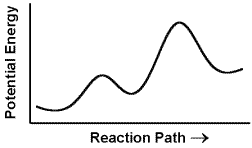A) 2 B) 4 C) 1 D) 3 E)  5
 6 In Question 5 the energy change for the overall reaction is
 A) exothermic B) endothermic C) zero D) impossible to tell
 7 Rates of most chemical reactions ______.
 A) are independent of temperature B) decrease with decreasing temperature C) decrease with increasing temperature8 The rate of the following reaction in water              S2O82-  + 2I-2SO42-  +  I2is found to double when the concentration of I- is doubled, and increases by a factor of 4 when both the concentration of I- and S2O82- are doubled.  The rate law for the reaction is
 A) rate = k [S2O82-] [I-]2 B) rate = k [I-]2 C) rate = k [S2O82-] [I-] D) rate = k [S2O82-] E) rate = k [I-]
 9 The reaction              2NO(g) + 2H2(g)N2(g) + 2H2O(g) obeys a rate law that is first order in H2 and second order in NO.  Find the correct statement:
 A) The overall order of the rate expression is 4. B) The rate of disappearance of H2 is equal to the rate of disappearance of NO. C) The rate of appearance of N2 is twice the rate of disappearance of NO. D) The overall order of the rate expression is 2.
10.

The rate of the reaction xX + yY + zZPRODUCTS has the following dependence on reactant concentrations:

 [X] [Y] [Z] rate 1 1 1 k 2 2 2 16k 1 1 2 2k 2 1 2 4k

The rate law for the reaction is

 A) rate = k[X]2[Y][Z] D) rate = k[X][Y]3[Z] B) rate = k[X][Y][Z] E) rate = k[X][Y][Z]2 C) rate =  k[Y]2[Z] F) rate = k[X][Y]2[Z]11 The half-life of the first order photodecomposition of the carcinogen nitrosodimethylamine is 30 min.  How many minutes will it take for 99.9% of a sample to decompose?
 A)  1784 B)  692 C)  299 D)  157 E)  93
12. Predict the rate of the gas reaction

2NO2 + O3N2O5  + O2

according to the proposed elementary mechanism:

 (1) NO2 + O3NO3 + O2 (slow) (2) NO3 + NO2N2O5 (fast)
 A) rate = k[NO2][O3] D) rate = k[NO2]2 B) rate = k[NO2][O3]2 E) rate = k[NO2] C) rate =k[NO2]2[O3]
 13 The rate constant of an elementary reaction A+BC+D is two-thirds the rate constant of the reverse reaction A+B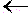C+D at the same temperature.  The correct statement is:
 A) The activation energy of the forward reaction is greater than the activation energy of the reverse reaction. B) The activation energy is the same in both directions, so the reverse reaction must be catalyzed while the forward reaction remains uncatalyzed. C) The activation energy of the reverse reaction is greater than the activation energy of the forward reaction.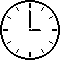14. The rate law for the elementary reaction mechanism

 (1) 2NO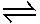N2O2 (rapid equilibrium) (2) N2O2 + O22N O2 (slow)

is expected to be

 A) first-order in NO and first-order in O2. B) second-order in NO and second-order in O2. C) second-order in NO and first-order in O2. D) first-order in NO and second-order in O2. E) second-order in NO and independent of O2. F) none of the above.
 15 A certain reaction for which the activation energy is 80 kJ/mole proceeds very slowly at 300K.  When the temperature is increased to 400K the rate constant of the reaction increases by a factor of
 A) 2 x 108 D) 6 x 105 B) 1 x 104 E) 3 x 103 C) 5 x 102
 16 What are the units of the rate constant k for a bimolecular reaction?
 A) (mols/liter)2 s-1 D) (mols/liter)-2 s-2 B) (mols/liter)-1 s-2 E) (mols/liter)-1 s-1 C) (mols/liter)-2 s-1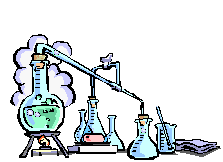17 Which rate law has an overall order of 1.5?
 A) rate = k[A][B][C]-1 D) rate = k[A]2[B]1/2 B) rate = k[A]2[B]1/2[C]-1 E) rate = k[A][B][C]1/2 C) rate = k[A][B]
18. A proposed mechanism for the decomposition of hydrogen peroxide is

 (1) H2O2 + I-H2O +  IO- (slow) (2) IO-  +  H2O2H2O + O2 +  I- (fast)

Find the statement that is false:

 A) The overall reaction is  2H2O22H2O + O2. B) The first step in the mechanism is the rate-determining step. C) I-  is a catalyst. D) IO-  is an intermediate. E) The rate expression is independent  of the concentration of I- .
19. Predict the rate of the reaction

Cl2 + COCOCl2

according to the proposed elementary mechanism:

 (1) Cl22Cl (rapid equilibrium) (2) Cl + COCOCl (rapid equilibrium) (3) COCl + Cl2COCl2 + Cl (slow)
 A) rate = k[Cl2][CO] D) rate = k[Cl2]1/2[CO] B) rate = k[Cl2]3/2[CO] E) rate = k[Cl2][CO]2 C) rate =k[Cl2]2[CO]
 20 The carbon-14 nucleus decays by beta emission according to a first-order rate law with a half-life of 5730 years.  How much of an initial sample of carbon-14 remains unreacted after 42,000 years?
 A)  0.92% B)  0.84% C)  0.75% D)  0.62% E)  0.49%